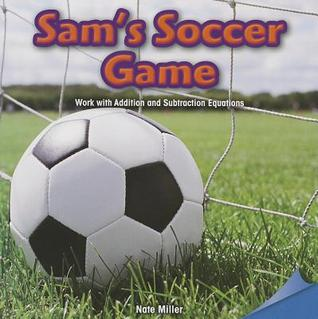Home » Sams Soccer Game: Work with Addition and Subtraction Equations by Nate Miller# Sams Soccer Game: Work with Addition and Subtraction Equations

## Nate Miller

Published August 1st 2013
ISBN : 9781477721896
Paperback
24 pages
Book Rating:Enter the sum

 About the Book Algebra is a foundational concept in the study of mathematics, and readers will delight in learning the principles of operations and algebraic thinking in a relatable and engaging way. Topics such as adding and subtracting within 20, using algebraicMoreAlgebra is a foundational concept in the study of mathematics, and readers will delight in learning the principles of operations and algebraic thinking in a relatable and engaging way. Topics such as adding and subtracting within 20, using algebraic principles to solve equations and word problems, and understanding the relationship between addition and subtraction will give beginning math students a firm grasp on basic math concepts. Sams soccer game is the backdrop for a lesson about the equal sign. Readers will be asked to determine if equations are true or false. This volume satisfies CCSS Math Standard 1.OA.D.7.